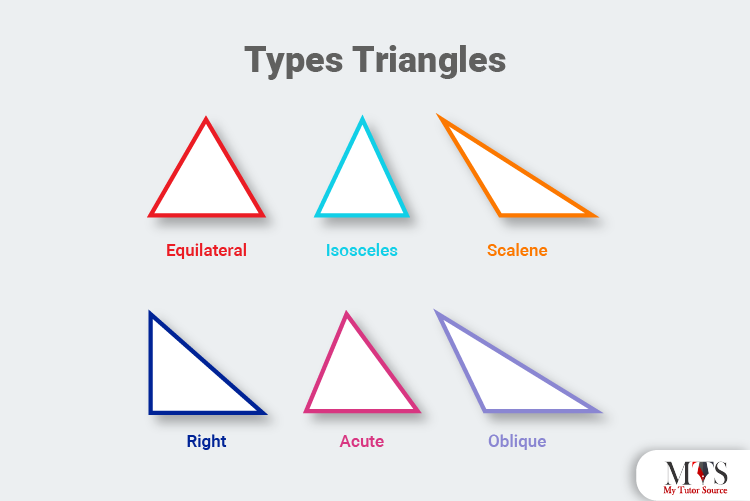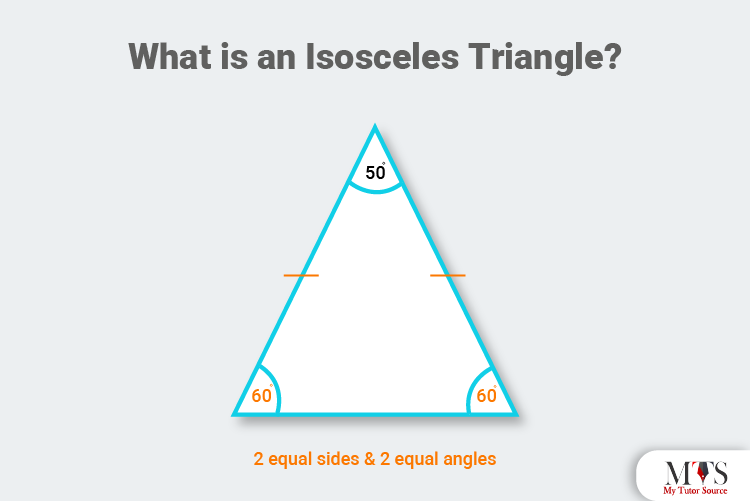# Triangles: What Are the Properties of an Isosceles Triangle?

• Published On:Triangles are one of the most used and one of the first shapes studied in geometry. Therefore, understanding the basic concepts, properties, type, angles, and every other detail is considered important by all the private math tutors and teachers around the globe. Interestingly, all types of triangles, such as the isosceles triangle or scalene, form out of straight-line segments with some angles and side length. Before discussing the isosceles triangles' properties, let us have a quick overview of the classification and angles of triangles.

## Types of Triangles

A two-dimensional geometric shape made up of three-line segments connected at their endpoints or vertices is known as a triangle. They have three vertices, three sides, three angles and are classified into three types according to the lengths of their sides, such as:### 1. Equilateral Triangle

All three sides are equal in length.

### 2. Scalene Triangle

All three sides are unequal in length.

### 3. Isosceles Triangle

Its two sides are equal in length.

## Angles of Triangles

### 1.  Acute Angle Triangle

A triangle with all of its interior angles less than 900 is known as an acute angle triangle.

### 2.  Obtuse Angle Triangle

A triangle with one interior angle of more than 900 is an obtuse angle triangle.

### 3.  Right Angle Triangle

A triangle with only one interior angle of 900 is known as a right-angle triangle.

## What is an Isosceles Triangle?

A polygon with at least two sides of equal length, angles, three vertices, and three edges is an isosceles triangle. The sum of the internal angle of an isosceles triangle is always equal to 1800.

Like the isosceles triangles, other triangles also differ based on their side lengths and angles as they own individual properties. For example, scalene, isosceles and equilateral triangles are defined based on their sides. On the other hand, right angled triangles, acute angle triangles, and obtuse angle triangles are classified on the basis of their angles.

Below is the figure of an isosceles triangle. Take a look before we move to its properties.## Properties of Isosceles Triangles

Each triangle has basic properties that make it unique from others. Here are a few characteristics and properties of an isosceles triangle:

• The unequal side of the isosceles triangle is known as the base, and the other two equal sides are known as the legs of the triangle.
• The angle between equal sides of an isosceles triangle is called the apex angle or vertex angle.
• The angles between the base of the isosceles triangle are always equal.
• Its perpendicular bisects the apex angle and base from the apex angle.
• Angles opposite to the equal sides of the isosceles triangle are congruent to each other. Two congruent base angles are also known as the isosceles triangle theorem (angle based).
• One or two isosceles triangles are always similar.
• The altitude from the apex divides the isosceles triangle into two congruent right-angled triangles.
• In a right-angled isosceles triangle, two angles are always 45 degrees, and the third is 90 degrees (right angle).
• The altitude on the hypotenuse of a right-angled isosceles triangle is half the length of the hypotenuse.
• A line segment or altitude that extends from the isosceles triangle's apex to its base's midpoint, is perpendicular to its base.
• The center of the circumcircle lies on the hypotenuse, and the radius of the circumcircle of the right-angled isosceles triangle is half the length of the hypotenuse.
• The altitude to the base is the line symmetry of the isosceles triangle.
• Three angles within the isosceles triangle are less than 90 degrees, which signifies acute angles.
• All three angles of this triangle add up to 180 degrees.

## Isosceles Triangle Formulas

• The area of isosceles triangle = ½ × Base × Height
• The perimeter of isosceles triangle = sum of its three sides

written as, P = 2a + b

• The altitude of isosceles triangle = h = √ (a2 − b2/4)

You can also find the area of the isosceles triangle using the following formulas:

• A = ½ [√ (a2 − b2 ⁄4) × b] with all three sides.
• A = ½ × b × c × sin(α) with the length of two sides and an angle between them
• A = [c2×sin(β)×sin(α) / 2×sin (2π−α−β)] with two angles and length between them.
• A = ½ × a2 to find area of an isosceles right triangle

Here,

a = length of two equal sides

b = base of the isosceles triangle

h = height of the isosceles triangle

## Real-Life Examples of the Isosceles Triangle

Numerous things around us and in the world are of isosceles triangle shape. Here are a few popular real-life examples of isosceles triangles:

• Pizza slice
• A pair of earnings

## Practice Questions of Isosceles Triangle

### Question: If an isosceles triangle's base angle is four more than twice the vertex angle. What would be the base angle?

Solution:

As all triangles are of 180 degrees

So,

Let x the vertex angle

And 2x+4 is the base angle

In mathematical form,

Vertex angle = x

Base angle = 2x + 4

Equation to find the vertex angle

⇒ x+(2x+4) + (2x+4) =180

⇒ 5x + 8 = 180

⇒ 5x = 172

⇒ x = 34.4

adding x value to find the base angle

⇒2x + 4

⇒2 (34.4) + 4

⇒ 72.8

Hence, vertex angle = 34.4 and the base angle = 72.8

### Question: If two equal sides of an isosceles triangle are 10 cm each base of 5 cm. what would be the perimeter?

Solution:

Using formula

Perimeter of an isosceles triangle = P = 2a + b

Here,

a = 10 cm

b = 5 cm

putting values in formula

P = 2 (10) + 5

P = 20 + 5

P = 25

Hence, the perimeter of a given triangle is approximately 25 cm.

### Question: With a side of 5 units and a base of 8 units, find out the area of an isosceles triangle?

Solution:

Using formula

A = b/4[ √ (4a2 - b2)]

Here,

a = 5

b = 8

by putting values

A = 8/4 [ √ (4 (5)2 – (8)2)]

A = 2 [ √ (4 (5)2 – (8)2)]

A = 2 [ √ (4 (25) – (64))]

A = 2 [ √ (100 – 64)]

A = 2 [ √ 36]

A = 2 (6)

A = 12

Hence, the area of the given isosceles triangle is 12 units2

• The term isosceles is derived from the ancient Greek word ‘ἰσοσκελής (isoskelḗs)’ and the Latin word ‘īsoscelēs,’ which means equal-legged.
• The isosceles-shaped buildings are earthquake-resistant.

### Find Top Tutors in Your AreaAustin has 10+ years of experience in teaching. He has researched on thousands of students-related topics, issues, and concerns. You will often find him writing about the common concerns of students, their nutrition, and what is beneficial for their academics and health both.